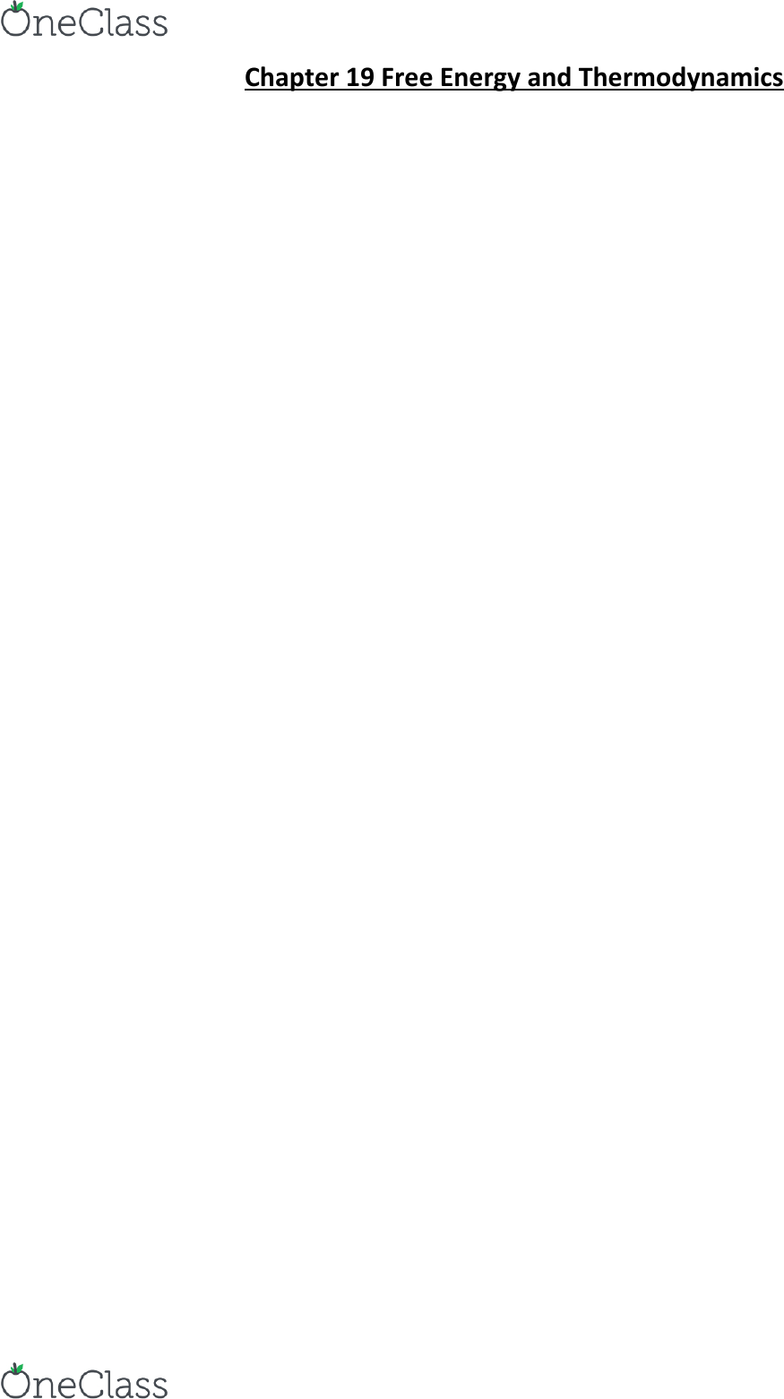Textbook Notes (290,000)
US (110,000)
UC-Irvine (3,000)
CHEM (200)
CHEM 1B (30)
Chapter 19

# CHEM 1B Chapter Notes - Chapter 19: Chemical Equation, Reversible Reaction, Spontaneous Process

Department
Chemistry
Course Code
CHEM 1B
Professor
Sergey Nizkorodov
Chapter
19

Page:
of 4Chapter 19 Free Energy and Thermodynamics
Energy spreads out or disperse from a more concentrated to a less concentrated form
Second Law of Thermodynamics simply states that Energy Spreads Out
19.2 Spontaneous and Nonspontaneous Processes
Spontaneous process = one that occurs without ongoing outside intervention
Don’t confuse spontaneity with speed of a reaction
Rate of a process can increase due to catalyst but catalyst cannot make a process spontaneous
A nonspontaneous reaction is not impossible
Nonspontaneous reaction can be made spontaneous by coupling with another spontaneous reaction
19.3 Entropy and the Second Law of Thermodynamics
Most spontaneous processes are exothermic, some spontaneous processes are endothermic
Entropy
oEntropy(S) = a thermodynamic function that increases with the number of energetically
equivalent ways to arrange the components of a system to achieve a particular state
oS klnW (k= Boltzmann constant = 1.38 * 10^-23J/K)
oMacrostate = A given set of conditions (P, V, and T) defines this of the system
oMicrostate = the exact internal energy distribution among the particles at any one instant
oW is the number of possible microstates that can result in a given macrostate
oGreater entropy= more energetically equivalent ways to arrange the components of the system
oThe state with the highest entropy also has the greatest dispersal of energy
oA state in which a given amount of energy is more highly dispersed (or more highly randomized)
has more entropy than a state in which the same energy is more highly concentrated.
The Second Law of Thermodynamics
oSecond law of Thermodynamics = For any spontaneous process, the entropy of the universe
increases ()
oSpontaneous process contribute to the entropy of the universe, Entropy = state function
o
oA chemical system proceeds in a direction that increases the entropy of the universe
Macrostates and Microstates
oClass Lecture Slides for Permutations of Microstates
find more resources at oneclass.com
find more resources at oneclass.com
The Units of Entropy
oEntropy is a measure of energy dispersal (joules) per unit temperature (kelvins)
19.4 Predicting Entropy and Entropy Changes for Chemical Reactions
- Structure determines properties
The Entropy Change Associated with a Change in State
oEntropy increases as it changes from solid to liquid or liquid to gas
oGas has the most ways to distribute its energy therefore greater entropy
The Entropy Change Associated with a Chemical Reaction ()
oStandard entropy change for a reaction() = the change in entropy for a process in which all
reactants and products are in their standard states
o
oStandard molar entropies () = used in calculating
Standard Molar Entropies (S) and the Third Law of Thermodynamics
oThird law of thermodynamics = The entropy of a perfect crystal at absolute zero (0K) is zero
Only has one possible way to arrange itself (W= 1)
oRelative Standard Entropies: Gases, Liquids, and Solids
Gas forms have more ways to arrange its components so greater energy dispersal
oRelative Standard Entropies: Molar Mass
Entropy increases with molar mass. More closely spaced = greater dispersal allowed
oRelative Standard Entropies: Allotropes
Allotropes = elements in two or more forms but different structures
Less constrained structure of graphite results in greater entropy
oRelative Standard Entropies: Molecular Complexity
Entropy increases with increasing molecular complexity
Molecules have greater entropy than free atoms
oRelative Standard Entropies: Dissolution
Dissolution of crystalline solid into solution results in increase of entropy
Calculating the Standard Entropy Change () for a Reaction
oTo calculate subtract the standard entropies of the reactions multiplied by their stoichiometric
coefficients from the standard entropies of the products multiplied by their stoichiometric
coefficients.
find more resources at oneclass.com
find more resources at oneclass.com
oStandard entropies are always nonzero at 25C
19.5 Heat Transfer and Entropy Changes of the Surroundings
-
- the release of heat energy by the system disperse that energy into the surroundings, increasing entropy
- An exothermic process increases the entropy of the surroundings
-An endothermic process decreases the entropy of the surroundings
The Temperature Dependence of
oThe greater the temperature the smaller the increase in entropy for a given amount of energy
oAt low temperatures, decrease in entropy is overcome by large increase in the entropy of sur
Quantifying Entropy Changes in the Surroundings
oA process that emits heat into surroundings increases the entropy of the surroundings
oProcess that absorbs heat from the surroundings, decreases the entropy of the surroundings
oThe magnitude of the change in entropy of the surroundings is proportional to the magnitude
of heat of the system, and inversely proportional to the temperature in kelvins
19.6 Gibbs Free Energy
-Gibbs free energy (G) =
- The change in Gibbs free energy for a process occurring at constant temperature and pressure is proportional
to the negative of
- decrease in Gibbs free energy ( < 0) corresponds to a spontaneous process
-increase in Gibbs free energy ( > 0) corresponds to a nonspontaneous process
The Effect of , and T on Spontaneity
oCase 1: Negative, S Positive
The reaction is spontaneous at all temperatures
find more resources at oneclass.com
find more resources at oneclass.com